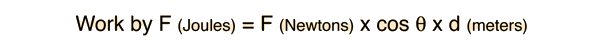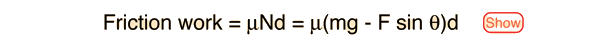# Work and the Work-Energy PrincipleEnter data in the boxes below. When you click outside the data box, the calculation will be made.

Start with a mass m of kg and an initial velocity v0= m/s.
Force angle = degrees and friction coefficient .Work = J= N x x m.Friction work = J= ( - )N x m.Final kinetic energy = J = Initial KEJ + Net workJ
From the final kinetic energy, the final velocity is v = m/s.

Note: This calculation presumes that the applied force F is not sufficient to lift the block off the surface. For forces large enough to lift the block, it will give negative work for friction, which is of course incorrect.

Index

Work-energy principle

 HyperPhysics***** Mechanics R Nave
Go Back